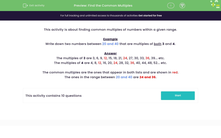# Find the Common Multiples

In this worksheet, students will find two common multiples of numbers within a given range.Key stage:  KS 2

Curriculum topic:   Number: Addition, Subtraction, Multiplication and Division

Curriculum subtopic:   Identify Common Factors, Multiples and Prime Numbers

Popular topics:   Division worksheets, Numbers worksheets

Difficulty level:#### Worksheet Overview

This activity is about finding common multiples of numbers within a given range.

Example

Write down two numbers between 20 and 40 that are multiples of both 3 and 4.

The multiples of 3 are:  3, 6, 9, 12, 15, 18, 21, 24, 27, 30, 33, 36, 39... etc.

The multiples of 4 are:  4, 8, 12, 16, 20, 24, 28, 32, 36, 40, 44, 48, 52... etc.

The common multiples are the ones that appear in both lists and are shown in red.

The ones in the range between 20 and 40 are 24 and 36.

You might have seen that 12 was a common multiple of 3 and 4, but, the question asked us to find common multiples between 20 and 40.

Remember, when we see the phrase: 'Write down two numbers between 20 and 40' ... this means that neither of these numbers can be our answer.

Between means numbers that can be found within these two points.

Does that all make sense?Then let's get started!

### What is EdPlace?

We're your National Curriculum aligned online education content provider helping each child succeed in English, maths and science from year 1 to GCSE. With an EdPlace account you’ll be able to track and measure progress, helping each child achieve their best. We build confidence and attainment by personalising each child’s learning at a level that suits them.

Get started